Open in App
Not now

# Reduce Data Dimensionality using PCA – Python

• Difficulty Level : Expert
• Last Updated : 18 Jul, 2022

## Introduction

The advancements in Data Science and Machine Learning have made it possible for us to solve several complex regression and classification problems.  However, the performance of all these ML models depends on the data fed to them. Thus, it is imperative that we provide our ML models with an optimal dataset. Now, one might think that the more data we provide to our model, the better it becomes – however, it is not the case. If we feed our model with an excessively large dataset (with a large no. of features/columns), it gives rise to the problem of overfitting, wherein the model starts getting influenced by outlier values and noise. This is called the Curse of Dimensionality.

The following graph represents the change in model performance with the increase in the number of dimensions of the dataset. It can be observed that the model performance is best only at an option dimension, beyond which it starts decreasing.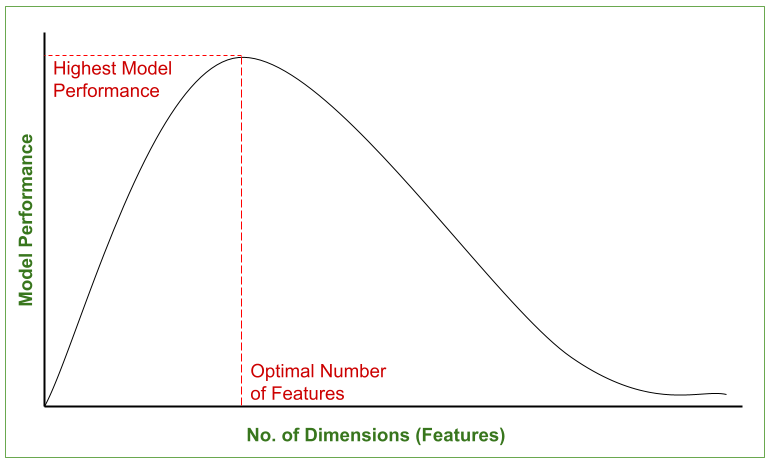Model Performance Vs No. of Dimensions (Features) – Curse of Dimensionality

Dimensionality Reduction is a statistical/ML-based technique wherein we try to reduce the number of features in our dataset and obtain a dataset with an optimal number of dimensions.

One of the most common ways to accomplish Dimensionality Reduction is Feature Extraction, wherein we reduce the number of dimensions by mapping a higher dimensional feature space to a lower-dimensional feature space. The most popular technique of Feature Extraction is Principal Component Analysis (PCA)

## Principal Component Analysis (PCA)

As stated earlier, Principal Component Analysis is a technique of feature extraction that maps a higher dimensional feature space to a lower-dimensional feature space. While reducing the number of dimensions, PCA ensures that maximum information of the original dataset is retained in the dataset with the reduced no. of dimensions and the co-relation between the newly obtained Principal Components is minimum. The new features obtained after applying PCA are called Principal Components and are denoted as PCi (i=1,2,3…n). Here, (Principal Component-1) PC1 captures the maximum information of the original dataset, followed by PC2, then PC3 and so on.

The following bar graph depicts the amount of Explained Variance captured by various Principal Components. (The Explained Variance defines the amount of information captured by the Principal Components).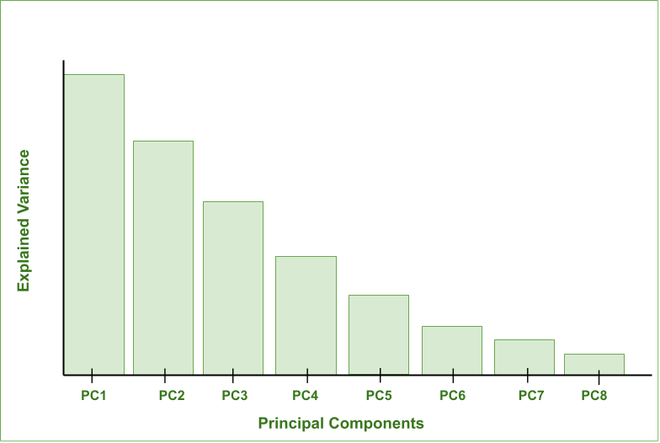Explained Variance Vs Principal Components

In order to understand the mathematical aspects involved in Principal Component Analysis do check out Mathematical Approach to PCA. In this article, we will focus on how to use PCA in Python for Dimensionality Reduction.

## Steps to Apply PCA in Python for Dimensionality Reduction

We will understand the step by step approach of applying Principal Component Analysis in Python with an example. In this example, we will use the iris dataset, which is already present in the sklearn library of Python.

Step-1: Import necessary libraries

All the necessary libraries required to load the dataset, pre-process it and then apply PCA on it are mentioned below:

## Python3

 `# Import necessary libraries` `from` `sklearn ``import` `datasets  ``# to retrieve the iris Dataset` `import` `pandas as pd  ``# to load the dataframe` `from` `sklearn.preprocessing ``import` `StandardScaler  ``# to standardize the features` `from` `sklearn.decomposition ``import` `PCA  ``# to apply PCA` `import` `seaborn as sns  ``# to plot the heat maps`

After importing all the necessary libraries, we need to load the dataset. Now, the iris dataset is already present in sklearn. First, we will load it and then convert it into a pandas data frame for ease of use.

## Python3

 `#Load the Dataset` `iris ``=` `datasets.load_iris()` `#convert the dataset into a pandas data frame` `df ``=` `pd.DataFrame(iris[``'data'``], columns ``=` `iris[``'feature_names'``])` `#display the head (first 5 rows) of the dataset` `df.head()`

Output: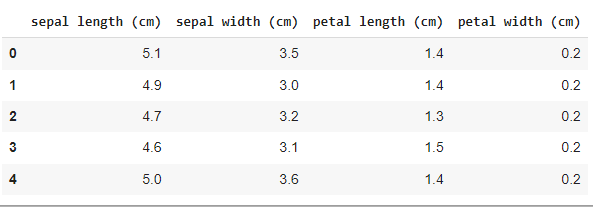iris dataset

Step-3: Standardize the features

Before applying PCA or any other Machine Learning technique it is always considered good practice to standardize the data. For this, Standard Scalar is the most commonly used scalar. Standard Scalar is already present in sklearn. So, now we will standardize the feature set using Standard Scalar and store the scaled feature set as a pandas data frame.

## Python3

 `#Standardize the features` `#Create an object of StandardScaler which is present in sklearn.preprocessing` `scalar ``=` `StandardScaler() ` `scaled_data ``=` `pd.DataFrame(scalar.fit_transform(df)) ``#scaling the data` `scaled_data`

Output: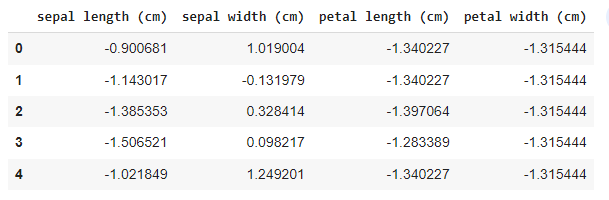Scaled iris dataset

Step-3: Check the Co-relation between features without PCA (Optional)

Now, we will check the co-relation between our scaled dataset using a heat map. For this, we have already imported the seaborn library in Step-1. The correlation between various features is given by the corr() function and then the heat map is plotted by the heatmap() function. The colour scale on the side of the heatmap helps determine the magnitude of the co-relation. In our example, we can clearly see that a darker shade represents less co-relation while a lighter shade represents more co-relation. The diagonal of the heatmap represents the co-relation of a feature with itself – which is always 1.0, thus, the diagonal of the heatmap is of the highest shade.

## Python3

 `#Check the Co-relation between features without PCA` `sns.heatmap(scaled_data.corr())`

Output: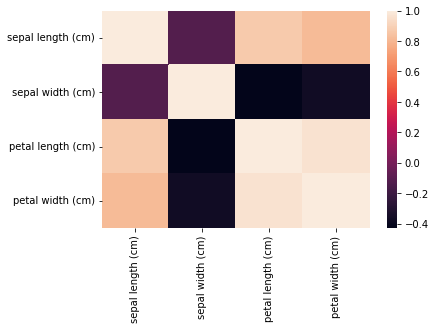Co-relation Heatmap of Iris dataset without PCA

We can observe from the above heatmap that sepal length & petal length and petal length & petal width have high co-relation. Thus, we evidently need to apply dimensionality reduction. If you are already aware that your dataset needs dimensionality reduction – you can skip this step.

Step-4: Applying Principal Component Analysis

We will apply PCA on the scaled dataset. For this Python offers yet another in-built class called PCA which is present in sklearn.decomposition, which we have already imported in step-1. We need to create an object of PCA  and while doing so we also need to initialize n_components – which is the number of principal components we want in our final dataset. Here, we have taken n_components = 3, which means our final feature set will have 3 columns. We fit our scaled data to the PCA object which gives us our reduced dataset.

## Python

 `#Applying PCA` `#Taking no. of Principal Components as 3` `pca ``=` `PCA(n_components ``=` `3``)` `pca.fit(scaled_data)` `data_pca ``=` `pca.transform(scaled_data)` `data_pca ``=` `pd.DataFrame(data_pca,columns``=``[``'PC1'``,``'PC2'``,``'PC3'``])` `data_pca.head()`

Output: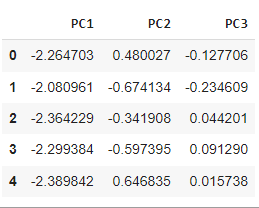PCA Dataset

Step-5: Checking Co-relation between features after PCA

Now that we have applied PCA and obtained the reduced feature set, we will check the co-relation between various Principal Components, again by using a heatmap.

## Python3

 `#Checking Co-relation between features after PCA` `sns.heatmap(data_pca.corr())`

Output: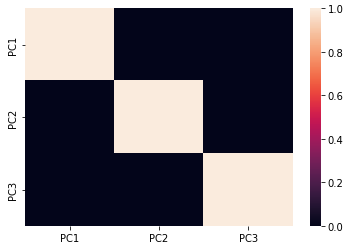Heatmap after PCA

The above heatmap clearly depicts that there is no correlation between various obtained principal components (PC1, PC2, and PC3). Thus, we have moved from higher dimensional feature space to a lower-dimensional feature space while ensuring that there is no correlation between the so obtained PCs is minimum. Hence, we have accomplished the objectives of PCA.

## Advantages of Principal Component Analysis (PCA):

1. For efficient working of ML models, our feature set needs to have features with no co-relation. After implementing the PCA on our dataset, all the Principal Components are independent – there is no correlation among them.
2. A Large number of feature sets lead to the issue of overfitting in models. PCA reduces the dimensions of the feature set – thereby reducing the chances of overfitting.
3. PCA helps us reduce the dimensions of our feature set; thus, the newly formed dataset comprising Principal Components need less disk/cloud space for storage while retaining maximum information.

My Personal Notes arrow_drop_up
Related Articles